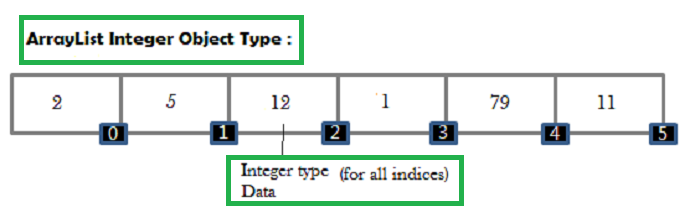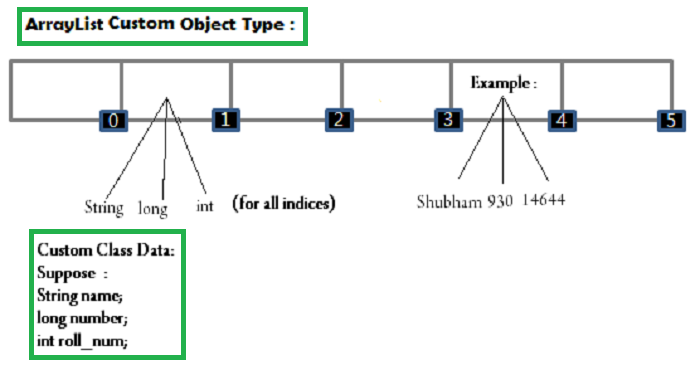# Custom ArrayList in Java

Prerequisite – ArrayList in Java
ArrayList in Java (equivalent to vector in C++) having dynamic size. It can be shrinked or expanded based on size. ArrayList is a part of collection framework and is present in java.util package.
An ArrayList:

```ArrayList <E> list = new ArrayList <> ();
```

E here represents an object datatype e.g. Integer. The Integer class wraps a value of the primitive type int in an object. An object of type Integer contains a single field whose type is int.Custom ArrayList: A custom arraylist has attributes based on user requirements and can have more than one type of data. This data is provided by a custom inner class which is formed by combination of various primitive object datatypes.

Consider a case when we have to take input as N number of students and details are:
roll number, name, marks, phone number
A normal method using object arraylist would be:

```   // define 4 ArrayLists and save data accordingly in
// each of them.

// roll number arraylist
ArrayList<Integer> roll = new ArrayList<>();

// name arraylist
ArrayList<String> name = new ArrayList<>();

// marks arraylist
ArrayList<Integer> marks = new ArrayList<>();

// phone number arraylist
ArrayList<Long> phone = new ArrayList<>();

// and for n students
for(int i = 0; i < n; i++)
{
// add all the values to each arraylist
// each arraylist has primitive datatypes
}
```

Now using a custom Arraylist:
The custom ArrayList simply supports multiple data in the way as shown in this image.To construct Custom ArrayList

• Build an ArrayList Object and place its type as a Class Data.
• Define a class and put the required entities in the constructor.
• Link those entities to global variables.
• Data received from the ArrayList is of that class type which stores multiple data.

 `// Java program to illustrate customArraylist in Java ` `import` `java.util.ArrayList; ` ` `  `class` `CustomArrayList ` `{ ` `    ``// custom class which has data type ` `    ``// class has defined the type of data ArrayList ` `    ``// size of input 4 ` `    ``int` `n=``4``; ` ` `  `    ``// the custom datatype class ` `    ``class` `Data ` `    ``{ ` `        ``// global variables of the class ` `        ``int` `roll; ` `        ``String name; ` `        ``int` `marks; ` `        ``long` `phone; ` ` `  `        ``// constructor has type of data that is required ` `        ``Data(``int` `roll, String name, ``int` `marks, ``long` `phone) ` `        ``{ ` `            ``// initialize the input variable from main ` `            ``// function to the global variable of the class ` `            ``this``.roll = roll; ` `            ``this``.name = name; ` `            ``this``.marks = marks; ` `            ``this``.phone = phone; ` `        ``} ` `    ``} ` ` `  `    ``public` `static` `void` `main(String args[]) ` `    ``{ ` `        ``// suppose the custom input data ` `        ``int` `roll[] = {``1``, ``2``, ``3``, ``4``}; ` `        ``String name[] = {``"Shubham"``, ``"Atul"``, ``"Ayush"``, ``"Rupesh"``}; ` `        ``int` `marks[] = {``100``, ``99``, ``93``, ``94``}; ` `        ``long` `phone[] = {8762357381L, 8762357382L, 8762357383L, ` `                        ``8762357384L ` `                       ``}; ` ` `  `        ``// Create an object of the class ` `        ``CustomArrayList custom = ``new` `CustomArrayList(); ` ` `  `        ``// and call the function to add the values to the arraylist ` `        ``custom.addValues(roll, name, marks, phone); ` `    ``} ` ` `  `    ``public` `void` `addValues(``int` `roll[], String name[], ``int` `marks[], ` `                          ``long` `phone[]) ` `    ``{ ` `        ``// local custom arraylist of data type ` `        ``// Data having (int, String, int, long) type ` `        ``// from the class ` `        ``ArrayList list=``new` `ArrayList<>(); ` ` `  `        ``for` `(``int` `i = ``0``; i < n; i++) ` `        ``{ ` `            ``// create an object and send values to the ` `            ``// constructor to be saved in the Data class ` `            ``list.add(``new` `Data(roll[i], name[i], marks[i], ` `                                              ``phone[i])); ` `        ``} ` ` `  `        ``// after adding values printing the values to test ` `        ``// the custom arraylist ` `        ``printValues(list); ` `    ``} ` ` `  `    ``public` `void` `printValues(ArrayList list) ` `    ``{ ` `        ``// list- the custom arraylist is sent from ` `        ``// previous function ` ` `  `        ``for` `(``int` `i = ``0``; i < n; i++) ` `        ``{ ` `            ``// the data received from arraylist is of Data type ` `            ``// which is custom (int, String, int, long) ` `            ``// based on class Data ` ` `  `            ``Data data = list.get(i); ` ` `  `            ``// data variable of type Data has four primitive datatypes ` `            ``// roll -int ` `            ``// name- String ` `            ``// marks- int ` `            ``// phone- long ` `            ``System.out.println(data.roll+``" "``+data.name+``" "` `                               ``+data.marks+``" "``+data.phone); ` `        ``} ` `    ``} ` `} `

Output:

```1 Shubham 100 8762357381
2 Atul 99 8762357382
3 Ayush 93 8762357383
4 Rupesh 94 8762357384
```

References:

This article is contributed by Shubham Saxena. If you like GeeksforGeeks and would like to contribute, you can also write an article using contribute.geeksforgeeks.org or mail your article to contribute@geeksforgeeks.org. See your article appearing on the GeeksforGeeks main page and help other Geeks.My Personal Notes arrow_drop_up
Article Tags :
Practice Tags :

7

Please write to us at contribute@geeksforgeeks.org to report any issue with the above content.